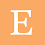## Saturday, January 31, 2015

### Problems and Solutions on Einstein's Photo Electric Equation

The phenomena of emission of electrons from a metal surface when light of suitable frequency incident on a metal surface is called photo electric effect.The incident light is a stream of photons and each photon has a fixed amount of energy.The incident energy is used to remove the electron from the metal surface and further will acquire some kinetic energy.This energy distribution is explained by Einstein  and the corresponding equation is called Einstein's photo electric equation.Here we are going to solve some problems basing on this concept.

Problem and solution

If a light of frequency 1.5 times threshold frequency incidents on metal surface, electrons are ejected with a certain velocity. If the incident frequency is halved and the intensity is doubled, what will be the velocity with which electrons are going to be ejected ?

The incident frequency shall be at least equal to threshold frequency for the emission of photo electrons. When the incident frequency is reduced to half which is just equal to threshold frequency, it becomes less than threshold frequency. And hence there is not going to be any photo electric effect. Increase of the intensity of light is not going to help here. It is simply because if you increase the intensity, more number of photons were ejected but each photon will have same frequency that is less than threshold frequency. Hence there is no photo electric effect in this case.

Problem and solution

The stopping potential of a metal surface is given as 9 V . What is the maximum speed of the rejected electron in this case?

We can solve this problem basing on the simple concept. When the electron is rejected from the cathode it will how kinetic energy and it will be moving towards the anode. When we apply reverse potentiality, at a particular potential photo electric current stops and that potentially is called stopping potential. So at the stopping potential, electron is having a energy which is equal to kinetic energy of the electron and opposite indirection. And hence both of them are able to be cancelled each other and their electron is getting stopped.

Taking this into consideration we can equate both the energies and solve the problem as shown in the first part of the given diagram.

Problem and solution

The page attached below is having another problem.

A graph is drawn taking the potential on x-axis and the photo electric current on y-axis. Three different situations were given and you are asked to identify, which of the following your options are correct?

You can analyse a question and comment at the end of the post for any kind of clarifications.

Problem and solution

A graph is drawn taking the applied potential on x-axis and the corresponding photo electric current on y-axis. Find the relation between the relation between the wavelengths of the incident light?

From the graph it is very clear that stopping potential for the second wavelength is more than that of the stopping potential of the first wavelength. We know that stopping potentially is directly proportional to incident frequency, when the incident frequency is more than the threshold frequency. Therefore as the second stopping potentially is more we can say the second incident frequency is more. As the frequency is reciprocal to wave length, second wavelength is less than that of the first wavelength.

Problem and solution

A graph is drawn taking the incident frequency on x-axis and the corresponding stopping potential on y-axis. What is the threshold wavelength of the light used ?

From the graph it is very clear that photo electric current starts from a certain frequency and that certain frequency is called the threshold frequency. Using the relation between velocity and the frequency of the light we can calculate the wavelength as shown below.

The above attached paper is having one more small problem.

Problem and Solution

If the velocity of the most energetic electron emitted is doubled when the incident frequency is doubled, what is the work function of the metal surface?

We can solve this problem basing on photo electric effect equation. According to the equation the incident energy is equal to sum of work function and the corresponding kinetic energy of the emitted electron. By applying the given data in that equation we can solve the problem as shown above.

Related Posts

#### 1 comment:

1.